# Philosophy, Computing, and Artificial Intelligence

PHI 319. The Computer Language Prolog.Thinking as Computation
Chapter 3 (41-61), Chapter 4 (63-81), Appendix B-C (279-288)
Prolog programs (in a zip file)

## Prolog ("PROgrammation en LOGique")

Prolog is a computer language based on logic programming. In this class (which is in philosophy, not computer science), we are not really interested in learning how to write Prolog programs. Our goal is to see that the backward chaining part of the logic programming/agent model can be implemented on a machine and so can be part of the "mind" of an artificial rational agent.

There are two environments in which to run Prolog programs. You can download SWI Prolog to run programs from the command line or use the online version to run programs in a web browser. The online version is easier for those without experience in computer programming.

### An Introduction to Prolog Programs and Queries

Learn Prolog Now! is a helpful (and gentle) tutorial. Prolog programs are definite logic programs. As such, they include clauses (facts and rules) and queries. On the left, there are two clauses written in the propositional calculus. On the right, there are the corresponding ways they are written in Prolog notation.

```
a               |               a.              (fact)
|
a ∨ ¬b ∨ ¬c     |               a :- b, c.      (rule) (head :- tail.)

```

The following set of equivalences (which we considered in the last lecture) helps to clarify the correspondence between the rule and a formula in the propositional calculus:

```
a ∨ ¬b ∨ ¬c      iff           a ∨ ¬(b ∧ c)
(b ∧ c) → a
a ← (b ∧ c)
a :- b, c.

```

Queries represent negative clauses. To see this, consider the following negative clause:

¬a ∨ ¬b ∨ ¬c

This negative clause is truth-functionally equivalent to

¬(a ∧ b ∧ c)

The prompt in the Prolog interpreter is

?-

So an initial query looks a little like a formula equivalent to a negative clause

?- a, b, c.

The basic evaluation step of Prolog is the backward chaining computation in logic programming that we considered in the previous lectures. Here is a query, program clause, and derived query:

```
In Prolog                               In logic

query           ?-a,d,e.                ¬a ∨ ¬d ∨ ¬e
program clause  a:-b,c.                  a ∨ ¬b ∨ ¬c
derived query   b,c,d,e.                ¬b ∨ ¬c ∨ ¬d ∨ ¬e

```

The derived query corresponds to the conclusion of an application of the deduction rule resolution. Given two clauses, Two literals are complimentary if and only if one is the negation of the other. resolution eliminates a pair of complementary literals and constructs a new clause (the resolvent) from the literals that remain.

In terms of the matching procedure, the query (?-a,d,e.) is processed last-in, first-out. So we search the KB top-down for clause whose head matches a. The match is with the clause (a:-b,c.). The tail (b,c) is pushed onto the query list to form the derived query (b,c,d,e.).

The relation of logical consequence that the evaluation step represents is

{negative clause, program clause} ⊢ negative clause

## A Screen Shot of Prolog in Action

This is not a course in computer programming, but you should play around some with Prolog.

Here (click the screenshot to enlarge it) is how the KB and the query look in the online version of Prolog: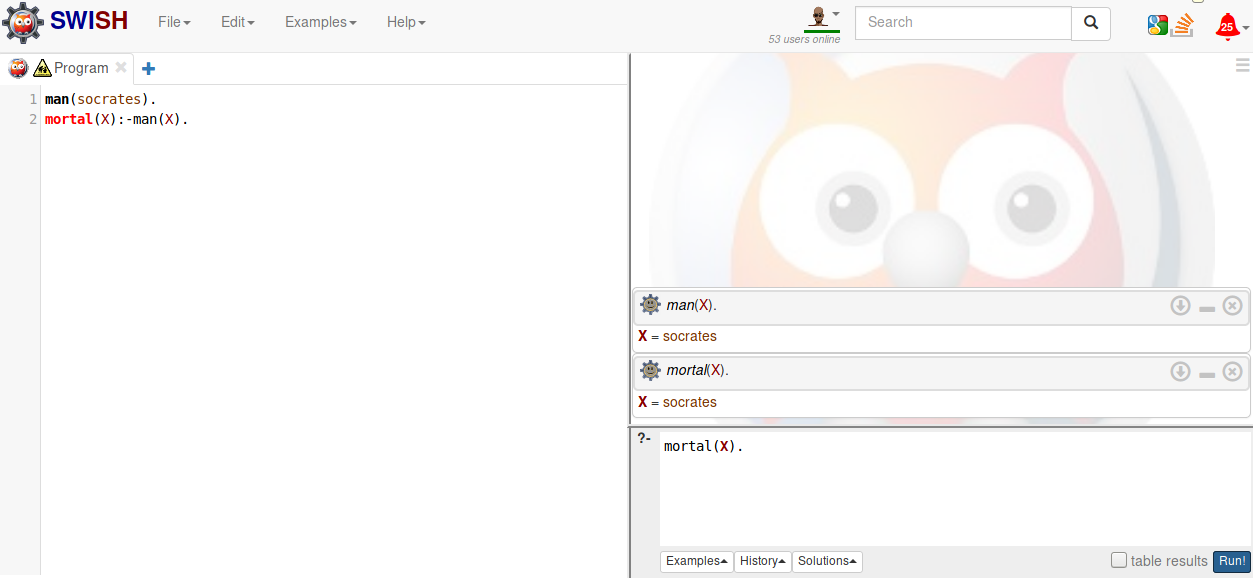Here is a screen shot of my terminal. I issue the command "swipl" to start the Prolog interpreter. In response, the interpreter issues the prompt (?-). This prompt signals that it is ready for a query. The query can be understood as giving the interpreter a goal. I give it the goal of adding a fact (that "Socrates is a man" ) and a rule (that "Everything that is a man is mortal") to its KB. The interpreter returns "true" to indicate that it is has satisfied the goal. Now the KB is

man(socrates).
mortal(X):-man(X).

Now I pose two queries ("something is mortal" and "something is a man").The queries give the interpreter the goal of determining whether the queries are logical consequences of the KB. Given the KB, Prolog should tell me that socrates is mortal and a man. That is what happens.

Prolog treats any term that begins with an uppercase letter (or the underscore) as a variable. So in the rule

mortal(X):-man(X),

Prolog treats X as a variable.

Because the variable was in the supplied goals (mortal(X) and man(X)) Prolog identifies the constant to which the variable is bound in the computation (X = socrates).

For explanation of the database manipulation commands in Prolog, see Database Manipulation in the tutorial.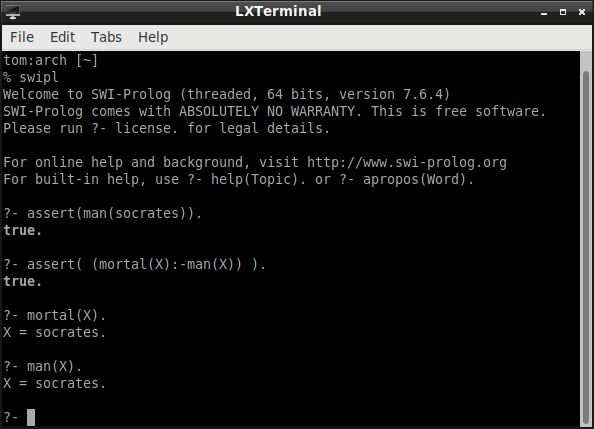## The "Family" Example (Thinking as Computation, 42)

I consult the file family.pl. This file contains the program:

child(john,sue). child(john,sam).
child(jane,sue). child(jane,sam).
child(sue,george). child(sue,gina).

male(john). male(sam). male(george).
female(sue). female(jane). female(june).

parent(Y,X) :- child(X,Y).
father(Y,X) :- child(X,Y), male(Y).
opp_sex(X,Y) :- male(X), female(Y).
opp_sex(Y,X) :- male(X), female(Y).
grand_father(X,Z) :- father(X,Y), parent(Y,Z)

I pose a few queries. I ask whether sue is the father of someone. I ask whether sam is the father of some female. I ask whether sam is the child of someone. Prolog can disregard the current binding and look for another. This is what happens when I type ";" (which is logically equivalent to "or"). The first reply is john. I use the symbol ; to ask whether sam is the child of someone else. I ask whether president_crow is male.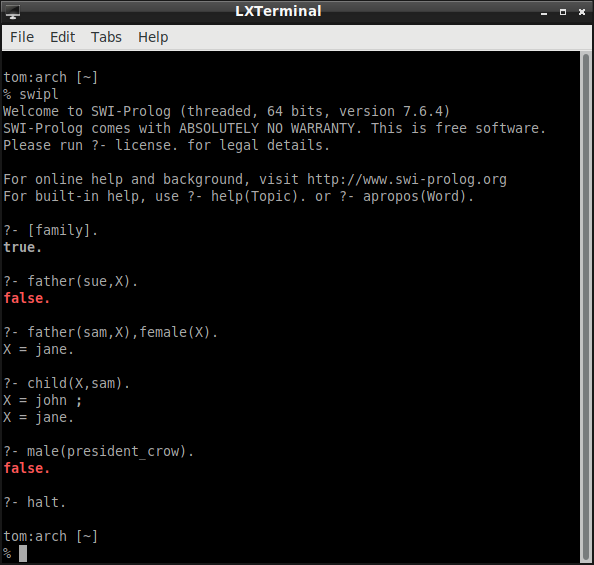To test your understanding, consider the last query and reply in the family example. President Crow (of ASU) is male, but the Prolog interpreter responds with "false."

What is going on here?

To answer, explain what "false" means in the reply. It does not mean that the proposition expressed by "President Crow is male" is false. It means that something else is false. What is it?

## The Pulp Fiction Example

I consult the file (I created called) pulpfiction.pl. This file contains the program. I list the two predicates in the program. I pose a few queries. I halt the Prolog interpreter.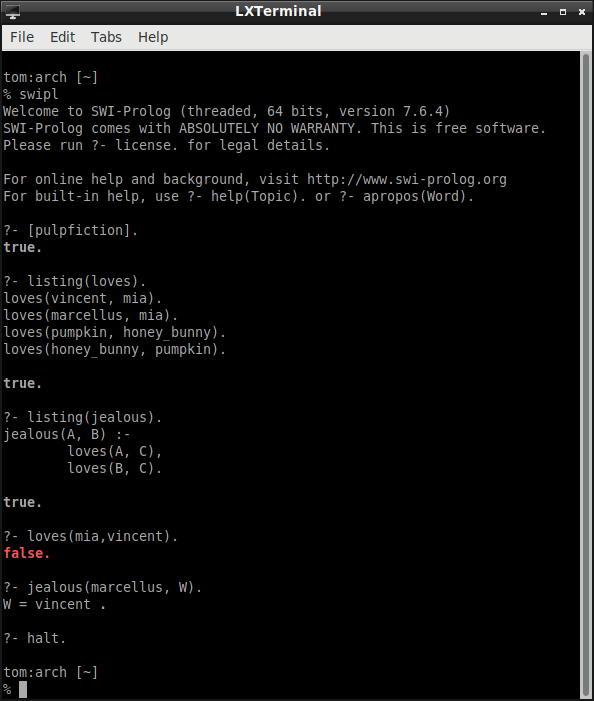## The "Blocks" Program

Here is the blocks program (blocks.pl) from the previous lecture:

on(b1,b2).
on(b3,b4).
on(b4,b5).
on(b5,b6).

above(X,Y) :- on(X,Y).
above(X,Y) :- on(X,Z), above(Z,Y).

This program consists in four facts (about which block is on top of which block) and two rules (that define the "above" relation). Corresponding to the facts and rules is the following model of the world (use your imagination to see blocks on top of one another):

```
b3
b4
b1     b5
b2     b6

```

Here is the trace for "above(b3, b5)."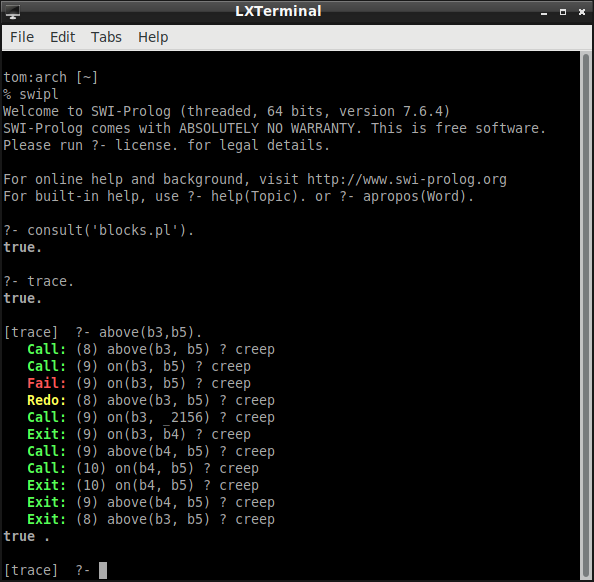creep causes the debugger to single-step. leap causes it to resume running the program. You probably thought computer scientists were without a sense of humor. The query "above(b3, b5)" unifies with the first rule. The derived query is "on(b3, b5)." This derived query fails. Now we have to try the query with the second rule.

The second rule now takes the form

above(b3, b5) :- on(b3, _2156), above(_2156, b5).

"_2156" is a variable. It is bound to "b4." The derived query list is "on(b3, b4), above(b4, b5)." These queries succeed, so the original query succeeds.

Here is the trace for "above(b3,b3)."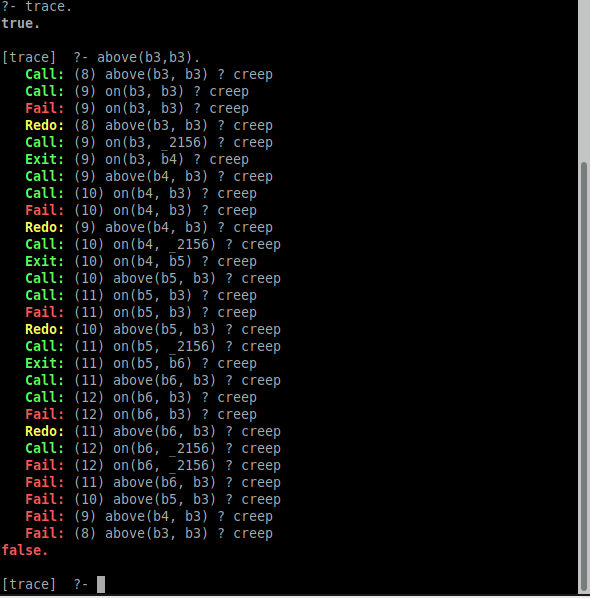The query "above(b3,b3)" is unified with the first rule "above(X,Y) :- on(X,Y)." This fails because "on(b3, b3)" fails. So the program tries to unify the query "above(b3,b3)" with the second rule "above(X,Y) :- on(X,Z), above(Z,Y)." This rule takes the form

"above(b3, b3) :- on(b3, _2156), above(_2156, b3)."

"_2156" is bound to "b4." The derived query list is "on(b3, b4), above(b4, b3)." The first query succeeds, but the second fails against the first rule because "on(b4, b3)" fails.

So now the program tries to unify "above(b4, b3)" with the second rule. This time the variable is bound to "b5." The derived query list is "on(b4, b5), above(b5, b3)." The first query in this list succeeds, but the second fails against the first rule because "on(b5, b3)" fails.

So now the program tries to unify "above(b5, b3)" with the second rule. This time the variable is bound to "b6." Now the derived query list is "on(b5, b6), above(b6, b3)." The first query in this list succeeds, but the second fails against the first rule because "on(b6, b3)" fails.

Now there is no constant to bind to the variable. So "above(b6, b3)" fails, "above(b5, b3)" fails, and "above(b4, b3)" fails. Hence the original query "above(b3, b3)" fails.

## Some Explanation of Lists in Prolog

We will uses lists in Prolog later in the course when we come to natural language processing (NLP). Lists are a finite sequence of elements. They are specified in Prolog by separating the elements by commas and enclosing them in square brackets. The empty list is a list.

A non-empty list has a head and a tail. The head is the first element. The tail is the rest. Prolog has a built-in operator | to decompose a list into its head and tail.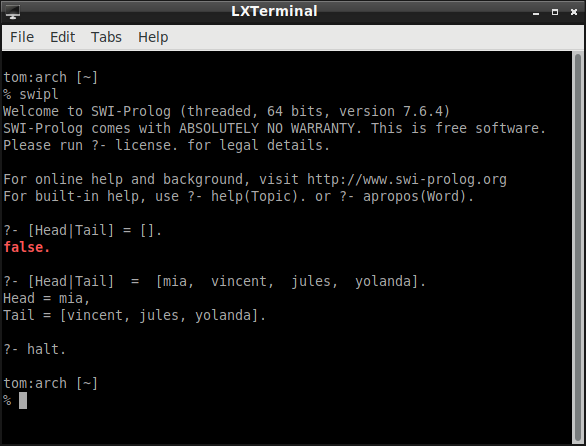## What we have Accomplished in this Lecture

We have become familiar with some of the basics of programming in Prolog and how Prolog incorporates some of the principles of logic programming.

This shows that machines can implement one form of intelligence. Given a set of premises and a query, they can answer positively if the query is a logical consequence of the premises.

This obviously does not solve "the problem of AI," but it is a step forward.